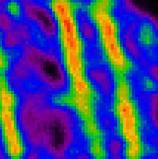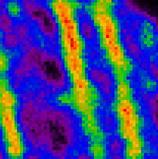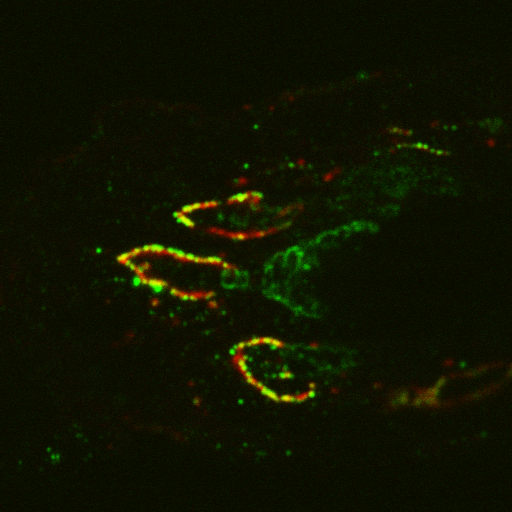# Signal-to-Noise ratio

## Introduction

The Signal-to-Noise ratio (SNR or S/N) is used in the Huygens Software as a Regularization Parameter, i.e. as a parameter that controls the sharpness of the restoration result. The higher this value, the sharper your restored image will be.

Therefore you should not think about the SNR as a parameter describing your original image, but as a tunable parameter that controls the deconvolved result, like the 'number of iterations' does.

Using a too large SNR value might be risky when restoring noisy originals, because you could be just enhancing the noise. A noise-free widefield image usually has SNR values higher than 50. A noisy confocal image can have values lower than 20.

## The SNR in the Essential Wizard

If you are doing deconvolution with the Huygens Essential deconvolution wizard, please see a practical approach in Set The Signal To Noise Ratio in the Essential Deconvolution Roadmap. The rest of this article give more details on this topic.

## Sample images for an estimation

Examples of SNR: same image, different SNR values... You can use this as a guide to visually estimate the SNR of your image.OriginalSNR = 30SNR= 15SNR = 5

## Definition

Throughout the Huygens Software the Signal-to-Noise ratio (SNR or S/N) of a digital microscopic image is defined as the square root of the number of photons in the brightest part of the image.

The idea behind this is that a Poisson Distribution is assumed for the Photon Noise. In this statistics, the "error" σ is the square root of the expectancy value P. Thus, the SNR would be

$$\text{SNR} = P / \sigma = \frac{P}{\sqrt{P}} = \sqrt{P}$$

If you know the conversion factor c (also called sometimes system gain) of your detector, calculating the SNR with the above definition is instant.

When you don't have this information, estimating the SNR is based on the idea of establishing the pixel value corresponding to a single photon 'hit'. Afterwards, one can calculate how many photons are involved in the most intense voxels of the image.

If you have Clipped Images you may not be able to properly determine the SNR, because the maximum or minimum intensities present in the image are not realistic.

### Not a background

Do not confound "noise" as defined here with other spurious signals, like the BackGround. To calculate the SNR you do not have to divide "signal" by "background", that would be something else (we can call that signal-to-background ratio SBR). Look at the sample images above: they clearly have different levels of noise, but the intensity averages in the high and low intensity regions are always the same, and therefore they have the same SBR.

The SNR measures the roughness or granularity of your image, and this is independent of the relation between signal and background.

## Estimation of the SNR

### Experimental measurement

The correct method to estimate the SNR must find the Standard Deviation (STD) around the maximum level, the "noise" around what is defined to be our "signal". Ideally, one would introduce in the sample a large homogeneous high intensity plateau, where (because of the present noise) some standard deviation would be measured.

Unfortunately this is experimentally difficult, and you never have a large volume with high constant intensities. The maximum in a real sample is usually very much localized (in some features of the objects) and intensity varies quickly around it, therefore you can't assume that its STD is due to noise only.

If you still imagine that you can do such experimental preparation, then the ratio between maximum (averaged) value and its STD would provide the SNR. (We focus on the number of photons, not on recorded intensity, but the two values are ideally related by a constant c, the camera conversion factor.)

The STD around the maximum intensity M in such 'calibration' plateau would be, in absence of other experimental artifacts, caused by the intrinsic Poisson statistics of the photon-electron events. And in such statistics, the STD is
(proportional to) the square root of the number of photons. This means that

$$\text{SNR} = \frac{ M }{\text{STD}} = \frac{c\ P}{c\ \sqrt{P}} = \sqrt{P}$$

Because this experimental approach is almost never possible, we need another more practical one.

Estimating the SNR in noisy images is surprisingly easy: we will outline the procedure in step-by-step form below. In low noise images it is much more difficult. Fortunately, in such cases the establishment of the precise SNR is not very important for the ICTM or MLE restoration method.

### The high noise case

Most dots in the background originate from single photon hits. Estimating the SNR is based on the idea to establish the pixel value corresponding to a single photon `hit'. In many microscopes the hits will not be located in a single pixel but instead distributed over two or three pixels adjacent in the fastest scanning direction, usually the x-direction. Follow these steps to compute the SNR:
• Zoom up the image in the Expand viewer (Slicer page) until you can clearly see individual pixels.
• Establish the value of the dark areas by pointing the cursor at these locations and reading off the voxel value in the Slicer page.
• Look for dots in the background with low intensity. There should be many dots with similar intensity, each of them the result of a single photon hit. In most cases the intensity of the photons will be spread over two or three adjacent voxels. Sum these values to get the total intensity of a single photon hit. Depending on the design of the data acquisition system you will see the spreading of the intensity in an asymmetric way (with a tail in the forward scan direction), or in a well designed system in a symmetric way.
• Establish the value of the voxel with maximum intensity, for example by asking Huygens for the image statistics.
• Subtract the Black Level from both the single hit intensity and the maximum intensity, to obtain the good values isingle and imax.
• Compute the number of photons in the maximum value voxel by dividing its intensity imax by the single hit intensity isingle. The S/N ratio is now:

$$\text{SNR} = \sqrt{ \frac{i_{max}}{i_{single}} }$$

### The low noise case

In low noise images you cannot see the individual photon hits anymore. Methods exists where the energy in the image outside the band limit (which can only be due to noise) is compared to the in-band energy. Other methods rely on information gathered during the averaging of single scan lines, and must therefore be integrated in the microscope image acquisition software. In principle one could establish the SNR accurately from a series of images (sections) taken at the same z-position.

Fortunately both the ICTM and MLE methods need only a rough guess of the true SNR. As a rule of thumb good quality confocal fluorescence images have a SNR between 20 to 40. Images recorded with a cooled CCD camera on top of a conventional microscope can easily have SNR ratios above 50, even above 100.

In the Deconvolution Recipes describing the use of the MLE and ICTM methods we outline a simple procedure to experiment with various settings of the SNR parameter.

## Direct determination of the SNR

The conversion factor c of your detector (e.g. a CCD camera) determines the number of electrons needed to increase the grey-value i of a VoXel a single level:

$$N_{electrons} = i c$$

(This factor c refers to electrons, not photons, because photons excite electrons in the detector that are actually "measured" by the electronics of the device. The conversion from photons to electrons is characterized by the quantum efficiency of the detector. An ideal detector, that accounts for all the captured photons, has a quantum efficiency of one. In any case we don't care here about the photons that were not detected, but only about those registered in the image.)

Given the factor c and the intensity value i_max of the brightest voxel in the image, the SNR is simply

$$\text{SNR} = \sqrt{ N_{electrons,max}\ } = \sqrt{ i_{max}\ c }$$

Intensity levels as reported by your detector (the i in the above equations) are sometimes said to be measured in ADU, analog-to-digital units, so the c would have ADU-1 units.

## Procedure for an improved estimation

In practice you start out with a SNR number derived either from an estimate of the number of photons in the image (as explained below), from rule of thumb reasoning (see the FAQ How to estimate the signal to noise ratio?), or from a visual inspection (see sample images above). Subsequently you do a restoration run and inspect the result for artifacts and residue background. If you are confident all is fine you rerun the restoration with a higher SNR setting (say 30%-50%) and perhaps a higher background. The software will continue were you left off. After this run you compare the results, for instance using the undo function (in Huygens Pro).

If you have done this a previous time for a similar image then of course you just use the values established then!!!

## Potential drawbacks of wrong estimations

The SNR number controls what you can think of as an artifact limiter. If you set it 'high', noise, if present, will be amplified. Also other distortions in the image formation might get amplified. A low SNR setting will attenuate these artifacts at the cost of the final resolution in your image.

If your estimation is too low, relevant information in your data will be considered to be noise and removed in the high-frequency regions: the resulting image will be too smooth, lacking details. See the FAQ What is the potential drawback of estimating the signal to noise ratio (SNR) to low? for more information.

If the estimation is too high, noise may not be properly removed and some artifacts generated. For example, a noisy background may lead to the appearence of tiny objects with certain structure in the restored image. See the following example:

• Raw image:• Deconvolved image with an overestimated SNR:Images (*) courtesy of J.A. Valentijn, Molecular Cell Biology Dept., Leiden University Medical Center.

Notice these green dim structures in the background, they probably are noise amplification only:For quantification of these effects, see Artifacts Or Features.

See the FAQ What is the potential drawback of estimating the signal to noise ratio (SNR) to high? for detailed information on the effect of SNR overestimation on the different Restoration Methods.

Read also http://www.photomet.com/library_enc_signal.shtml and http://www.photomet.com/library_enc_detect.shtml (Photometrics).

(*) The raw image was acquired with a Bio-Rad 2100MP confocal/multi-photon system (multi-photon option was not used here). The image was taken from a 7 micrometer thick cryostat section of rat colon tissue that was fixed in 4% paraformaldehyde. The section was labeled with rabbit antibodies against the small GTP-binding protein, rab3D, and secondary Alexa-488 goat anti-rabbit antibodies (green channel), and with Alexa-594 labeled Griffonia simplicifolia II lectin. Each V-shaped fluorescent structure represents the Golgi apparatus of a goblet cell (the mucus secreting epithelial cells in intestine). Images used with permission.